Swarthmore College

Engineering Methodology

The Nonlinear Pendulum

In Week 1, we introduced Matlab and showed you how to plot simple graphs of functions (vectors) in Matlab. In Week 2, you are to learn about Matlab functions and use a numerical integration routine to solve an equation that is unsolvable analytically. As before, if you feel unchallenged by this assignment, feel free to work on the slightly more difficult problem in italics at the end.

Problem

A pendulum consisting of a mass M connected to a massless string of length L hangs from a pivot under the influence of gravity, as shown in Figure 1. Using a free body diagram of the mass, derive the differential equation that governs the motion of the mass if it is initially displaced an angle q0 from its vertical (equilibrium) position at time t=0 and released. First derive an analytical solution assuming small angles. Then solve the differential equation numerically using the Matlab function ode23 and plot the angle q as a function of time, along with its time-derivative, in one graph window using the subplot command. Try small initial values of q0 as well as large ones, for comparison with the small-angle analytical solution.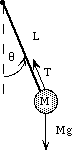Figure 1

Solution

The tension T in the string exerts no torque on the pivot (why not?), but the component of the weight vector in the direction perpendicular to the string does. The torque due to the weight is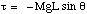(1)

The rotational analog of Newton's Second Law is t = Ia, where I is the moment of inertia equal to ML2 for a compact pendulum bob, and a is the angular acceleration given by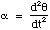(2)

Newton's Second Law therefore becomes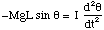(3)

or with I = ML2,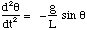with q = q0 at t = 0 andat t= 0 (4)

as the initial conditions. This is a nonlinear differential equation that in general cannot be solved analytically (although you will do it numerically). If you assume small angles, however, sin q = q and Equation 4 becomes one you can solve analytically: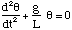(same i.c.'s as Eq. 4) (5)

The analytical solution is q(t) = A cos(wt), with an angular frequency w that you should verify is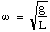(6)

and which is related to the period T (not to be confused with the tension) and the frequency f by the relationships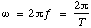(7)

To solve the problem in Matlab™, you will first need to separate a second-order differential equation into two first-order differential equations by a change of variables. Let

u1 = q and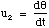(8)

then Equation 4 can be written as the two coupled equations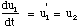(9)

and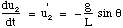(10)

This is known as the "state variable form" of the equation, and the initial conditions become u1 = q0 for the Eq. 9 and u2 = 0 for Eq. 10. These can be solved by creating a function in Matlab that outputs u1' and u2' for given values of u1 and u2. Once you have written such a function (see p. 434), a call to the ode23 function (see p. 140 and 509) will integrate the function numerically over the interval of time that you specify (20 s is good for L = 1 m), creating two arrays (the first is elapsed time) that you can plot using the subplot command. Please print out two pairs of graphs, one for large angles and one for small angles (you may choose your own constants).

Challenge assignment

Assume the pendulum can move anywhere in space (not just in the plane of the paper), consistent with the string being under tension. Given initial angles q and j with the (vertical) z-axis and initial horixontal velocities, trace the two-dimensional trajectory that would result from the subsequent motion. Hint: the same equations as those in Eq. 4 apply but they act independently in the x- and y- directions.